Courses

# Ex 10.6 NCERT Solutions- Circles Class 9 Notes | EduRev

## Class 9 Mathematics by Full Circle

Created by: Full Circle

## Class 9 : Ex 10.6 NCERT Solutions- Circles Class 9 Notes | EduRev

The document Ex 10.6 NCERT Solutions- Circles Class 9 Notes | EduRev is a part of the Class 9 Course Class 9 Mathematics by Full Circle.
All you need of Class 9 at this link: Class 9

Question 1. Prove that the line of centres of two intersecting circles subtends equal angles at the two points of intersection.
Solution:
We have two circles with centres O and O' respectively such that they intersect each other at P and Q.
To prove that ∠OPO' = ∠OQO', let us join OP, O'P, OQ, O'Q and OO'.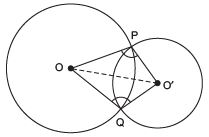In ΔOPO' and ΔOQO', we have OP = OQ               [Radii of the same circle]
O'P= O'Q               [Radii of the same circle]
OO' = OO'               [Common]
∴ Using the SSS criterion, ΔOPO'≌ ΔOQO'
∴ Their corresponding parts are equal.
Thus, ∠OPO' = ∠OQO'

Question 2. Two chords AB and CD of lengths 5 cm and 11 cm respectively of a circle are parallel to each other and are on opposite sides of its centre. If the distance between AB  and CD is  6 cm, find the radius of the circle.
Solution:
We have a circle with centre O. (Chord AB) || (Chord CD) and the perpendicular distance between AB and CD is 6 cm and AB = 5 cm, CD = 11 cm.

Let ‘r’ be the radius of the circle.
Let us draw OP ⊥ AB and OQ ⊥ CD.
We join OA and OC.
Let OQ = x cm
∴ OP = (6 ∠ x) cm               [∵ PQ = 6 cm]
∵ The perpendicular from the centre of a circle to chord bisects the chord.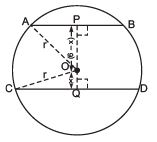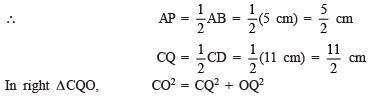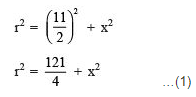in right Δ APO,    AO=  AP2 + OP2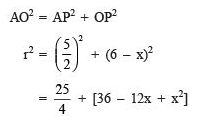...(2)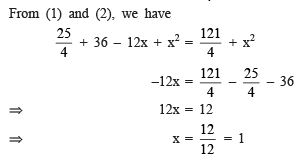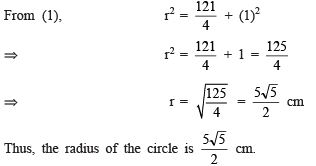Question 3. The lengths of two parallel chords of a circle are 6 cm and 8 cm. If the smaller chord is at distance 4 cm from the centre, what is the distance of the other chord from the centre?

REMEMBER

• The longer chord in a circle is nearer to the centre than the smaller chord.

Solution: We have a circle with centre O. Parallel chords AB and CD are such that the smaller chord is 4 cm away from the centre.
Let us draw OP ⊥ AB and join OA and OC.
∴ OP ⊥ AB
∴ P is the mid-point of AB.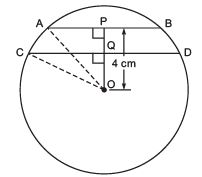⇒ AP = (1/2)AB = (1/2)(6 cm) = 3 cm
Similarly, CQ = (1/2)CD = (1/2)(8 cm) = 4 cm
Now in right ΔOPA, we have OA2 = OP2 + AP2
⇒ r2 = 42 + 32
⇒ r2 = 16 + 9 = 25
⇒ r=√25 = 5 cm
Note: r2 = 25
⇒ r = ±5 But we reject r = ∠5, because distance cannot be negative.
Again, in right ΔCQO, we have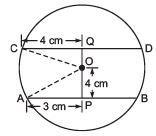OC2 = OQ2 + CQ2
⇒ r2 = OQ2 + 42
⇒ OQ2 = r∠ 42
⇒ OQ2 = 52 ∠ 4= 25
∠ 16 = 9               [∵ r = 5 cm]
⇒ OQ =√9= 3 cm
The distance of the other chord (CD) from the centre is 3 cm.
Note: In case we take the two parallel chords on either side of the centre, then
In ΔPOA, OA2 =OP2 + PA2r2 =42 + 3= 52
⇒ r = 5 cm
In ΔQOC, OC2 =CQ2 + OQ2
⇒ r2 =42 + OQ
⇒ OQ2 = 52 - 42 = 9
⇒ OQ = 3 cm

Question 4. Let the vertex of an angle ABC be located outside a circle and let the sides of the angle intersect equal chords AD and CE with the circle. Prove that ∠ABC is equal to half the difference of the angles subtended by the chords AC and DE at the centre.
Solution:
We have an ∠ABC such that the arms BA and BC on production, make two equal chords AD and CE. Let us join AC, DE and AE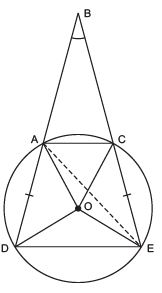∵ An exterior angle of a triangle is equal to the sum of interior opposite angles.
∴ In ΔBAE, we have Exterior ∠DAE = ∠ABC + ∠AEC               …(1)
∵ The chord DE, subtends ∠DOE at the centre and ∠DAE in the remaining part of the circle.

∴ ∠DAE = (1/2)∠DOE …(2)
Similarly, ∠AEC =(1/2)∠AOC               …(3)
From (1), (2) and (3), we have (1/2)∠DOE = ∠ABC + (1/2)∠AOC
⇒ ∠ABC = (1/2)∠DOE - (1/2)∠AOC
⇒ ∠ABC = (1/2) [∠DOE - ∠AOC]
⇒ ∠ABC = (1/2) [(Angle subtended by the chord DE at the centre) ∠ (Angle subtended by the chord AC at the centre)]
⇒ ∠ABC =(1/2) [Difference of the angles subtended by the chords DE and AC]

Question 5. Prove that the circle drawn with any side of a rhombus as diameter, passes through the point of intersection of its diagonals.
Solution:
We have a rhombus ABCD such that its diagonals AC and BD intersect at O.
Taking AB as diameter, a circle is drawn. Let us draw PQ || AD and RS || AB, both passing through O. P, Q, R and S are the mid-points of DC, AB, AD and BC respectively. ∵ All the sides of a rhombus are equal.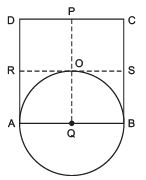∴ AB = DC
⇒   (1/2) AB =(1/2) DC
⇒ AQ = DP
⇒ BQ = DP
⇒ AQ = BQ               [∵ Q is the mid-point of AB]                 ...(1)
Similarly, RA = SB
⇒ RA = QO               [∵ PQ is drawn parallel to AD]               …(2)
From (1) and (2),
we have QA = QB = QO
i.e. A circle drawn with Q as centre, will pass through A, B and O.
Thus, the circle passes through the point of intersection (O) of the diagonals of the rhombus ABCD.

Question 6. ABCD is a parallelogram. The circle through A, B and C  intersect CD (produced if necessary) at E. Prove that AE = AD.
Solution:
We have a parallelogram ABCD. A circle passing  through A, B and C is drawn such that it intersects CD at E.
∵ ABCE is a cyclic quadrilateral.
∴ ∠AEC + ∠B = 180º               …(1)
[∵ Opposite angles of a cyclic quadrilateral are supplementary]
But ABCD is a parallelogram.               [Given]
∴ ∠D= ∠B               …(2)
[∵ Opposite angles of a parallelogram are equal]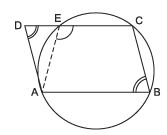From (1) and (2), we have ∠AEC + ∠D = 180º               …(3)
But ∠AEC + ∠AED = 180º               …(4)
From (3) and (4), we have ∠D= ∠AED
i.e. The base angles of ΔADE are equal.
∴ Opposite sides must be equal.

Question 7. AC and BD are chords of a circle which bisect each other. Prove that (i) AC and BD are diameters, (ii) ABCD is a rectangle.
Solution:
We have a circle with centre at O. Two chords AC and BD are such that they bisect each other. Let their point of intersection be O.
Let us join AB, BC, CD and DA.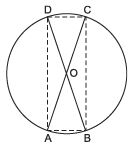(i) To prove that AC and BD are the diameters of the circle.
In ΔAOB and ΔCOD, we have
AO = OC               [∵ O is the mid-point of AC]
BO = DO               [∵ O is the mid-point of BD]
∠AOB = ∠COD               [Vertically opposite angles]
∴ Using the SAS criterion of congruence,
ΔAOB ≌ ΔCOD
⇒ AB = CD
⇒ arc AB = arc CD               ...(1)
Similarly, arc AD = arc BC               ...(2)
Adding (1) and (2), arc AB + arc AD = arc CD + arc BC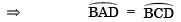⇒ BD divides the circle into two equal parts.
∴ BD is a diameter.
Similarly, AC is a diameter.

(ii) To prove that ABCD is a rectangle.
ΔAOB ≌ ΔCOD
⇒ ∠OAB = ∠OCD
⇒ ∠BAC = ∠ABD               (c.p.c.t)
⇒ AB || CD
∴ ABCD is a parallelogram.
Since, opposite angles of a parallelogram are equal.
∴ ∠DAB = ∠DCB
But ∠DAB + ∠DCB = 180º               [∵ Sum of the opposite angles of a cyclic quadrilateral is 180º]
⇒ ∠DAB = 90º  = ∠DCB
Thus, ABCD is a rectangle.

Question 8. Bisectors of angles, A, B and C of a triangle ABC intersect its circumcircle at D, E and F respectively. Prove that the angles of the triangle DEF are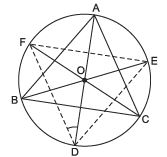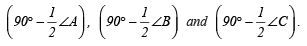Solution: We have a triangle ABC inscribed in a circle, such that bisectors of ∠A, ∠B and ∠C intersect the circumcircle at D, E and F respectively.
Let us join DE, EF and FD.
∵ Angles in the same segment are equal.
∴ ∠FDA = ∠FCA               …(1)
∠EDA = ∠EBA               …(2)
Adding (1) and (2), we have ∠FDA + ∠EDA = ∠FCA + ∠EBA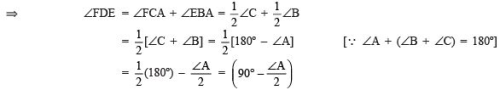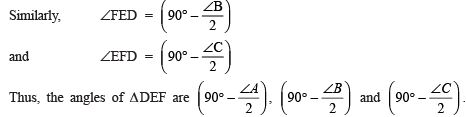Question 9. Two congruent circles intersect each other at points A and B. Through A any line segment PAQ is drawn so that P, Q lie on the two circles. Prove that BP = BQ.
Solution:
We have two congruent circles such that they intersect each other at A and B. A line passing through A, meets the circle at P and Q. Let us draw the common chord AB.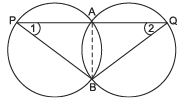∵ Angles subtended by equal chords in the congruent circles are equal.
∴ ∠APB = ∠AQB
Now, in ΔPBQ, we have ∠1= ∠2               [Proved]
∴ Their opposite sides must be equal.
⇒ PB = BQ

Question 10. In any triangle ABC, if the angle bisector of ∠ A and perpendicular bisector of BC intersect, prove that they intersect on the circumcircle of the triangle ABC.
Solution:
We have a ΔABC inscribed in a circle. The internal bisector of ∠A and the bisector of BC intersect at E.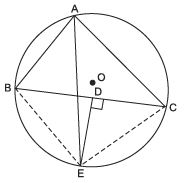Let us join BE and CE.
∵ ∠BAE = ∠CAE                       [∵ AE is the bisector of ∠ BAC]
∴ arc BE = arc EC
⇒ chord BE = chord EC
In ΔBDE and ΔCDE, we have BE = CE
BD = CD                        [Given]
DE = DE                        [Common]
∴ By SSS criterion of congruence,
ΔBDE ≌ ΔCDE
⇒ Their corresponding parts are equal.
∴ ∠BDE = ∠CDE
But ∠BDE + ∠CDE = 180º                        [Linear pairs]
⇒ ∠BDE = ∠CDE = 90º i.e.
DE ⊥ BC Thus, DE is the perpendicular bisector of BC.

132 docs

,

,

,

,

,

,

,

,

,

,

,

,

,

,

,

,

,

,

,

,

,

;